# Easiest colleges to get into in Arizona

Top 7 colleges in Arizona with the highest acceptance rates
What are the easiest colleges to get into in Arizona? We've got you covered. We've compiled a national college database and have created a list of the easiest colleges to get into in Arizona below. These are the easiest 4 year colleges to get into in Arizona that have the highest acceptance rates, and are more likely to accept low GPAs, GEDs, and everything in between. There are tons of good colleges below that accept more applicants, and we also share their average SAT and ACT scores so that you can see where you're most competitive. Read on to find out more.

## Arizona Western College acceptance rate

Arizona Western College acceptance rate is 100%.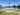The average SAT score for Arizona Western College is 1300.

• SAT composite: 1300
• SAT math: 650

The average ACT score for Arizona Western College is 28.

## Arizona State University acceptance rate

Arizona State University acceptance rate is 84.8%.The average SAT score for Arizona State University is 1250.

• SAT composite: 1250
• SAT math: 630

The average ACT score for Arizona State University is 26.

## University of Arizona acceptance rate

University of Arizona acceptance rate is 84.4%.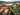The average SAT score for University of Arizona is 1196.

• SAT composite: 1196
• SAT math: 610

The average ACT score for University of Arizona is 25.

## University of Advancing Technology acceptance rate

University of Advancing Technology acceptance rate is 83%.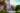The average SAT score for University of Advancing Technology is 1035.

• SAT composite: 1035
• SAT math: 510

The average ACT score for University of Advancing Technology is 21.

## Northern Arizona University acceptance rate

Northern Arizona University acceptance rate is 82.6%.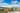The average SAT score for Northern Arizona University is 1130.

• SAT composite: 1130
• SAT math: 560

The average ACT score for Northern Arizona University is 23.

## Prescott College acceptance rate

Prescott College acceptance rate is 79.6%.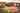The average SAT score for Prescott College is 1150.

• SAT composite: 1150
• SAT math: 550

The average ACT score for Prescott College is 24.

## Grand Canyon University acceptance rate

Grand Canyon University acceptance rate is 67%.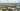The average SAT score for Grand Canyon University is 1000.

• SAT composite: 1000
• SAT math: 500